# Optimization in 1-D and N-D. Debugging Functions¶

## Unit 11, Lecture 1¶

Numerical Methods and Statistics

## Goals:¶

1. Learn the meaning of root-finding and minimization, the two types of optimization
2. Understand the iterative nature of these methods
3. Debug common problems when defining functions, which is essential for optimization
4. Be able to identify convex problems and understand their complexties
5. Learn the two standard methods for minimize and root-finding and how to call them in Python
In :
import numpy as np
import matplotlib.pyplot as plt


# Numerical Optimization - Root Finding¶

What is $x$ in

$$\cos (x) = x$$

To use a root-finding method, we must make our equation have one side be 0.

$$\cos (x) - x = 0$$

# Newton's Method - For finding Roots¶

In :
from scipy.optimize import newton

root =  newton(lambda x: np.cos(x) - x, x0=0)
print(root, np.cos(root) - root)

0.7390851332151606 1.1102230246251565e-16

$$x_{i+1} = x_i - \frac{f(x_i)}{f'(x_i)}$$Source: Wikipedia, Newton's Method

This method, like all this unit are iterative. This is modifiable by you, either through choosing tolerance, or maximum iterations. The functions have sensible defaults

In :
root =  newton(lambda x: np.cos(x) - x, x0=0, tol=1e-3)
print(root, np.cos(root) - root)

0.73908511214521 3.526292269295794e-08

In :
root =  newton(lambda x: np.cos(x) - x, x0=0, tol=1e-3, maxiter=5)
print(root, np.cos(root) - root)

0.73908511214521 3.526292269295794e-08


# Newton's Method¶

Use root instead, this is just for instructional purposes. root is better

Type: Root Finding

Discrete/Continuous: Continuous

Dimensions: 1

Derivative: optional

Non-Convex: not recommended

Python: newton

Let's try to solve a more interesting equation with Newton's method!

$$\int_0^x e^{-s^2}\,ds = \frac{1}{2}$$

Find $x$

In :
#Note: this code is intentionally really bad
def equation(x):
return quad(lambda s: np.exp(-s**2), 0, x)
root = newton(equation, x0=0)

---------------------------------------------------------------------------
TypeError                                 Traceback (most recent call last)
<ipython-input-5-b5fda6a0acf0> in <module>
3 def equation(x):
4     return quad(lambda s: np.exp(-s**2), 0, x)
----> 5 root = newton(equation, x0=0)

~/miniconda3/lib/python3.7/site-packages/scipy/optimize/zeros.py in newton(func, x0, fprime, args, tol, maxiter, fprime2, x1, rtol, full_output, disp)
316         q1 = func(p1, *args)
317         funcalls += 1
--> 318         if abs(q1) < abs(q0):
319             p0, p1, q0, q1 = p1, p0, q1, q0
320         for itr in range(maxiter):

TypeError: bad operand type for abs(): 'tuple'

# Steps for Debugging Code¶

1. Correct any Python errors
2. Restart the Kernel
3. Correct any Python errors
4. Print to discover logical errors

## Most Common Mistakes¶

1. Copy-Pasta leaving in old variables
2. Mixing Numpy arrays and for loops
3. Forgetting order of function args and return values

# Review of Functions¶

For functions like quad, newton, and minimize the function should take in 1 argument and return 1 argument.

# Correct Examples¶

$$x^2 - 3x + 2$$
In :
def version_1(x):
return x**2 - 3 *x + 2

version_2 = lambda x: x ** 2 - 3 *x + 2

np_version = np.vectorize(version_1)

print(version_1(3.))
print(version_2(3.))

some_threes = np.zeros(10)
some_threes[:] = 3.0 # -> Notice how we don't replace the numpy array with 3, but instead make all elements in it equal to three
#some_threes = 3 -> This would delete the numpy array and now some_threes would be a single 3

print(np_version(some_threes))

2.0
2.0
[2. 2. 2. 2. 2. 2. 2. 2. 2. 2.]

$$\int_{\pi}^x \sin^2(s)\,ds$$
In :
from scipy.integrate import quad
import numpy as np
from math import pi

def integrate_sin2(x):
ans, err = quad(lambda s: np.sin(s) ** 2, pi, x)
return ans

print(integrate_sin2(2 * pi))

1.5707963267948966


2 Vectors, $\vec{x}$ and $\vec{y}$, where one component of $\vec{x}$ is changing and we want to know the distance between the two vectors.

In :
from math import sqrt

def distance(x, y):
sum_sq = np.sum((x - y)**2)
return sqrt(sum_sq)

def my_distance(s):
x = np.zeros(3)
y = np.zeros(3)
x = 2.0
x = s
x = -3.5
y = 1.0
y = -3.0
y = 0.0
return distance(x, y)

print(my_distance(1))

5.408326913195984


## Incorrect Examples¶

### No Return Value¶

In :
def version_1(x):
x**2 - 3 *x + 2

print(version_1(3.))

None


In :
def integrate_sin2(x):
return quad(lambda s: np.sin(s) ** 2, pi, x)

print(integrate_sin2(2 * pi))

(1.5707963267948966, 1.743934249004316e-14)


### Too many arguments¶

In :
def distance(x, y):
sum_sq = np.sum((x - y)**2)
return sqrt(sum_sq)

print(distance(1))

---------------------------------------------------------------------------
TypeError                                 Traceback (most recent call last)
<ipython-input-11-6fd995bbf352> in <module>
3     return sqrt(sum_sq)
4
----> 5 print(distance(1))

TypeError: distance() missing 1 required positional argument: 'y'

$$\int_0^x e^{-s^2}\,ds = \frac{1}{2}$$

Find $x$

In :
#note still wrong
def equation(x):
ans, err = quad(lambda s: np.exp(-s**2), 0, x)
return ans
root = newton(equation, x0=0)
print(root)

0.0

In :
equation(root)

Out:
0.0

We forgot to rearrange the equation to be equal to $0$

In :
root = newton(lambda x: equation(x) - 0.5, x0=0)
print(root, equation(root))

0.5510394276090265 0.4999999999999999


## Scope¶

Scope means the set of variables and functions which are defined and accessible in your code

In :
x = 4
y = 2

#Right now, there is x,y and all other functions/variables defined or imported above in the scope


## Scopes nest¶

In :
x = 4
y = 2
#Here, I have x and y in scope
def scope_example():
z = 2
#Here, I have x,y and z in scope

#Here I again have only x and y in scope

In :
x = 4
y = 2
print(y,"Before function")
def scope_example():
y = 25 #This is a new version of y that exists only in this scope
print(y, "Inside function")
scope_example()
print(y, "After Function")

2 Before function
25 Inside function
2 After Function

In :
x = 4
y = 
print(y,"Before function")
def scope_example():
y = 25 #Here I'm not creating a y, but modifying y
print(y, "Inside function")
scope_example()
print(y, "After Function")

 Before function
 Inside function
 After Function


1. Scopes nest, so that you can access things above your scope
2. You can modify variables from any scope you can see, but ones you create disappear outside of the scope

# Applications of Optimization¶

1. Solving non-linear equations
2. Solving systems of equations
3. Optimizing equations with or without constraints
4. Fitting models to data

## Choosing which method to use¶

There are five things to consider when doing optimization:

1. Is it 1 or N dimensions?
2. Are you minimizing or root-finding?
3. Are there constraints?
4. Are there bounds?
5. Is it convex or non-convex?

# Identifying Convexity¶

If a problem has more than one minimum (derivative is 0), then the problem is non-convex. The opposite of convex is non-convex. A concave function can be made convex with a negative sign. A non-convex function cannot be made convex.

Knowing about convexity can come from:

• Plots
• Running convex optimization in two starting positions and getting different minimums
• Knowing something specific about the problem

Consider a function with two minimums:

In :
def two_well(x):
if x < 0.125:
return (x + 2) ** 2
if x >= 0.125:
return (x - 2) ** 2 + 1

np_two_well = np.vectorize(two_well)

In :
x = np.linspace(-4, 4, 1000)
plt.plot(x, np_two_well(x))
plt.show()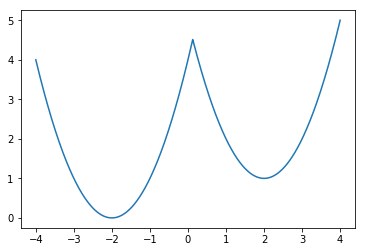# BFGS Optimization - Minimization¶

Broyden–Fletcher–Goldfarb–Shanno

# BFGS¶

Type: Minimization

Discrete/Continuous: Continuous

Dimensions: N

Derivative: optional

Non-Convex: not recommended

Python: minimize if no constraints or bounds are given

## Nomenclature¶

In optimization, you have a function called the objective function. That's what you're minimizing. It always returns a single value. It is sometimes called the fit, the error, the residual, or the penalty.

In :
def obj(x):
return x**2

x = np.linspace(-1,1,100)
plt.plot(x, obj(x))
plt.show()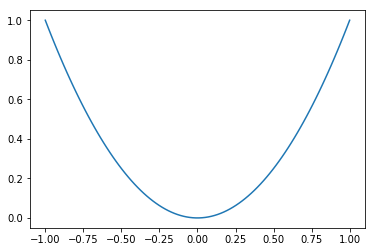In :
from scipy.optimize import minimize

minimize(obj, x0=3)

Out:
      fun: 2.5388964258140254e-16
hess_inv: array([[0.5]])
jac: array([4.67689909e-08])
message: 'Optimization terminated successfully.'
nfev: 9
nit: 2
njev: 3
status: 0
success: True
x: array([1.59339149e-08])

## Minimize Return value¶

     fun: The value of the function at the minimum
hess_inv: The inverse of the the Hessian
jac: The value of the Jacobian
message: A string describing what happened
nfev: Number of function evaluations
nit: Number of iterations of the x point
njev: Number of times it computed the Jacobian
status: The single digit message (0 = success, != 0 some error)
success: Boolean indicating success
x: The minimum x

# Objective Functions¶

Be careful that your objective function is convex and it's minimum isn't at $\infty$ or $-\infty$.

## A good objective function¶

Minimize the following:

$$f(x) = \frac{(x - 4)^2}{2} + \frac{(x - 2)^2}{4}$$

In :
def f(x):
return ((x-4)**2)/2+((x-2)**2)/4
x = np.linspace(-10, 10, 100)
plt.plot(x, f(x))
plt.show()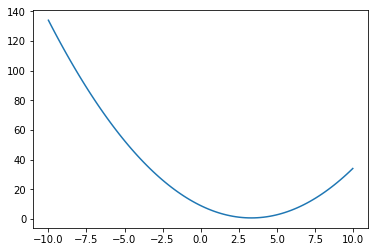In :
result = minimize(f, x0=0)
print(f'the minimum value occurs at {result.x} and is {result.fun}')

the minimum value occurs at [3.33333336] and is 0.6666666666666674


Minimize the following:

$$f(x) = \frac{(x - 4)} { 2 }$$

The minimum is at $-\infty$!

In :
result = minimize(lambda x: (x - 4) / 2, x0=0)
print(f'the minimum value occurs at {result.x} and is {result.fun}')

the minimum value occurs at [-1.7895697e+08] and is -89478487.25


## A good objective function but bad x0¶

Minimize the following function

$$4 \left[ r^{-12} - r^{-6}\right]$$
In :
result = minimize(lambda r: 4 * (r**(-12) - r**(-6)), x0=0)
print(f'the minimum is at {result.x}')

the minimum is at [0.]

/home/whitead/miniconda3/lib/python3.7/site-packages/ipykernel_launcher.py:1: RuntimeWarning: divide by zero encountered in power
"""Entry point for launching an IPython kernel.
/home/whitead/miniconda3/lib/python3.7/site-packages/ipykernel_launcher.py:1: RuntimeWarning: invalid value encountered in subtract
"""Entry point for launching an IPython kernel.


Our initial value was not in the domain!

In :
r = np.linspace(0.9, 2, 100)
y = 4 * (r**(-12) - r**(-6))
plt.plot(r, y)
plt.show()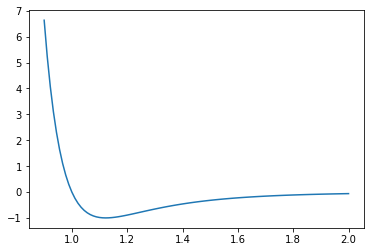In :
result = minimize(lambda r: 4 * (r**(-12) - r**(-6)), x0=1)
print(f'the minimum is at {result.x} and its value is {result.fun}')

the minimum is at [1.12246208] and its value is -0.9999999999999768


## Maximizing¶

In order to maximzie a function, just add a minus sign

Maximize the following function:

$$-\left[x - \cos(x)\right]^2$$
In :
#place - sign to make it a maxmimization problem
result = minimize(lambda x: (x - np.cos(x))**2, x0=1)
print(f'the maximum is at {result.x}')

the maximum is at [0.7390852]


## Multiple Dimensions¶

Just indicate multiple dimensions by using a multidimensional x0. Note that your $x$ becomes a vector!

Minimize the following:

$$f(x, y) = \frac{(x - 4)^2} { 2 } + \frac{(y + 3)^2} { 5 }$$
In :
result = minimize(lambda x: (x - 4)**2 / 2 + (x + 3)**2 / 5, x0=[0,0])
print(f'maximum occurs when x = {result.x} and y = {result.x}')

maximum occurs when x = 3.999999680451366 and y = -3.0000083683518133


## Identifying Convexity for Minimization - Example¶

The best ways to identify convexity are:

• plot it
• try optimizing in multiple starting positions
In :
x = np.linspace(-1, 1, 1000)
plt.plot(x,  2 * x ** 3 - 0 * x **2  - x)
plt.show()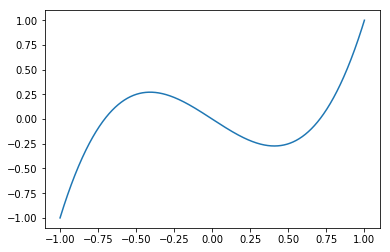In :
from scipy.optimize import minimize
minimize(lambda x:  2 * x ** 3 - 0 * x **2  - x, x0=0.05)

Out:
      fun: -0.27216552697590846
hess_inv: array([[0.20398928]])
jac: array([-1.11758709e-08])
message: 'Optimization terminated successfully.'
nfev: 24
nit: 5
njev: 8
status: 0
success: True
x: array([0.40824828])
In :
x = np.linspace(-1, 1, 1000)
plt.plot(x,  2 * x ** 3 - 0 * x **2  - x)
plt.show()In :
from scipy.optimize import minimize
minimize(lambda x:  2 * x ** 3 - 0 * x **2  - x, x0=-0.5)

Out:
      fun: -1.146240476075504e+25
hess_inv: array([])
jac: array([0.])
message: 'Optimization terminated successfully.'
nfev: 48
nit: 1
njev: 16
status: 0
success: True
x: array([-1.78956955e+08])

# Powell hybrid method¶

Type: Root finding

Discrete/Continuous: Continuous

Dimensions: N

Derivative: optional

Non-Convex: not recommended

Python: root unless method argument specifies a different method

## 1D Example¶

This is exactly like newton from above. Solve this equation:

$$\cos x + \sin x = x$$
In :
from scipy.optimize import root
#rearranged equation so all terms on one side
result = root(lambda x: np.cos(x) + np.sin(x) - x, x0=1)
print(result)

    fjac: array([[-1.]])
fun: array([2.22044605e-16])
message: 'The solution converged.'
nfev: 8
qtf: array([-2.42716958e-12])
r: array([1.64467312])
status: 1
success: True
x: array([1.25872818])


The result type is like what we saw for minimize. Similar terms are here, including the root and the value of the function at the root. Notice it's not exactly $0$ at the root.

In :
x = result.x
print(np.cos(x) + np.sin(x) - x)

[2.22044605e-16]


Solve the following system of equations: $$3 x^2 - 2 y = 4$$ $$x - 4 y ^ 2 = -2$$

In :
def sys(v):
#I'm using v here to distinguish from the x in the equations
#extract x and y
x = v
y = v
#compute equations
eq1 = 3 * x ** 2 - 2 * y - 4
eq2 = x - 4 * y**2 + 2
#pack into list
sys_eq = [eq1, eq2]
return sys_eq
root(sys, x0=[1,1])

Out:
    fjac: array([[-0.99129609, -0.13165126],
[ 0.13165126, -0.99129609]])
fun: array([2.87769808e-13, 3.16324744e-12])
message: 'The solution converged.'
nfev: 10
qtf: array([4.70588464e-09, 2.08104163e-08])
r: array([-8.45721872,  2.95677276,  7.06016501])
status: 1
success: True
x: array([1.39555277, 0.92135129])

So the answer is $x = 1.40,\: y = 0.921$

You should not ignore the information in the output of root. Imagine this small modification:

$$3 x^2 - 2 y^2 = 4$$$$x^3 - 4 y = -2$$
In :
def sys2(v):
#I'm using v here to distinguish from the x in the equations
#extract x and y
x = v
y = v
#compute equations
eq1 = 3 * x ** 2 - 2 * y**2 - 4
eq2 = x**3 - 4 * y + 2
#pack into list
sys_eq = [eq1, eq2]
return sys_eq
root(sys2, x0=[1,1])

Out:
    fjac: array([[-0.79311064, -0.60907759],
[ 0.60907759, -0.79311064]])
fun: array([-0.31652211,  0.4128055 ])
message: 'The iteration is not making good progress, as measured by the \n  improvement from the last ten iterations.'
nfev: 30
qtf: array([-3.93523929e-04, -5.20186955e-01])
r: array([-1.16194697e+01,  6.56882367e+00, -1.16411656e-03])
status: 5
success: False
x: array([1.53592084, 1.30262823])

If you did not read the message or status, you might believe the method succeeded.

More compact version:

In :
root(lambda x: [3 * x ** 2 - 2 * x - 4, x - 4 * x ** 2 + 2], x0=[1,1])

Out:
    fjac: array([[-0.99129609, -0.13165126],
[ 0.13165126, -0.99129609]])
fun: array([2.87769808e-13, 3.16324744e-12])
message: 'The solution converged.'
nfev: 10
qtf: array([4.70588464e-09, 2.08104163e-08])
r: array([-8.45721872,  2.95677276,  7.06016501])
status: 1
success: True
x: array([1.39555277, 0.92135129])

## Importance of starting position¶

By the way, there are two roots to this function!

In :
root(lambda x: [3 * x ** 2 - 2 * x - 4, x - 4 * x ** 2 + 2], x0=[0, 0])

Out:
    fjac: array([[ 0.98296038,  0.18381755],
[-0.18381755,  0.98296038]])
fun: array([ 2.66453526e-15, -4.44089210e-16])
message: 'The solution converged.'
nfev: 16
qtf: array([ 2.87224221e-12, -7.25968027e-13])
r: array([ 5.41242052, -0.44135572,  6.9600742 ])
status: 1
success: True
x: array([ 0.87635927, -0.84799164])